Скачать презентацию Urban and Regional Economics Part II The Structure

b4a22a386a170c754c1597669ae44495.ppt

• Количество слайдов: 44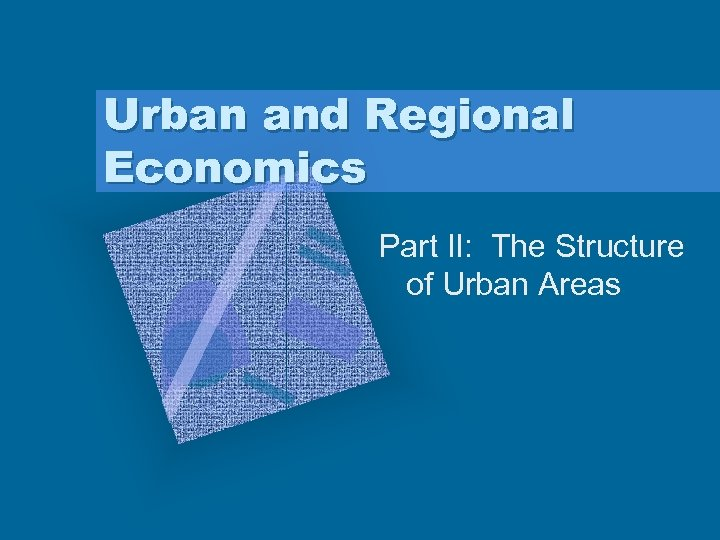Urban and Regional Economics Part II: The Structure of Urban Areas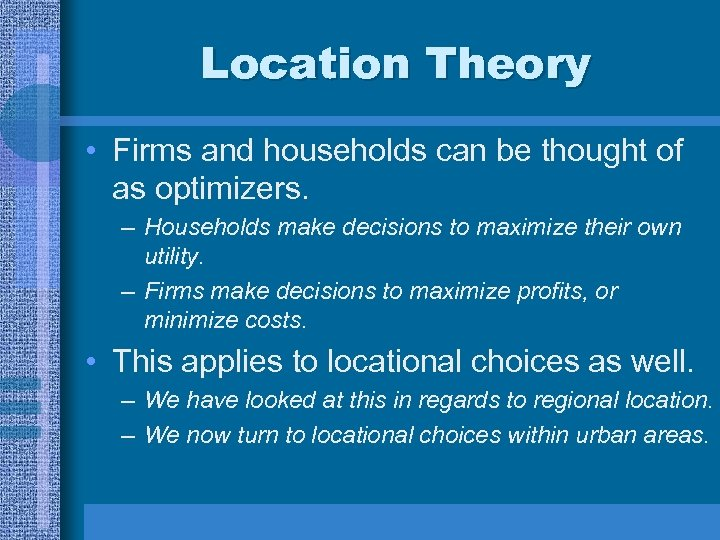Location Theory • Firms and households can be thought of as optimizers. – Households make decisions to maximize their own utility. – Firms make decisions to maximize profits, or minimize costs. • This applies to locational choices as well. – We have looked at this in regards to regional location. – We now turn to locational choices within urban areas.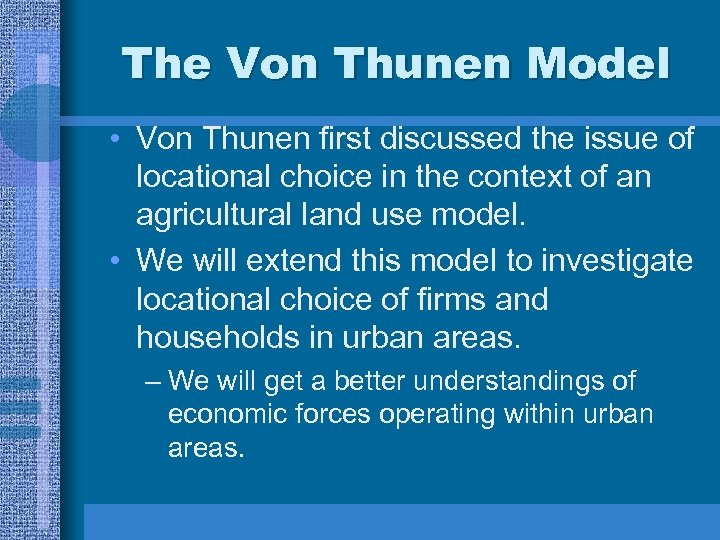The Von Thunen Model • Von Thunen first discussed the issue of locational choice in the context of an agricultural land use model. • We will extend this model to investigate locational choice of firms and households in urban areas. – We will get a better understandings of economic forces operating within urban areas.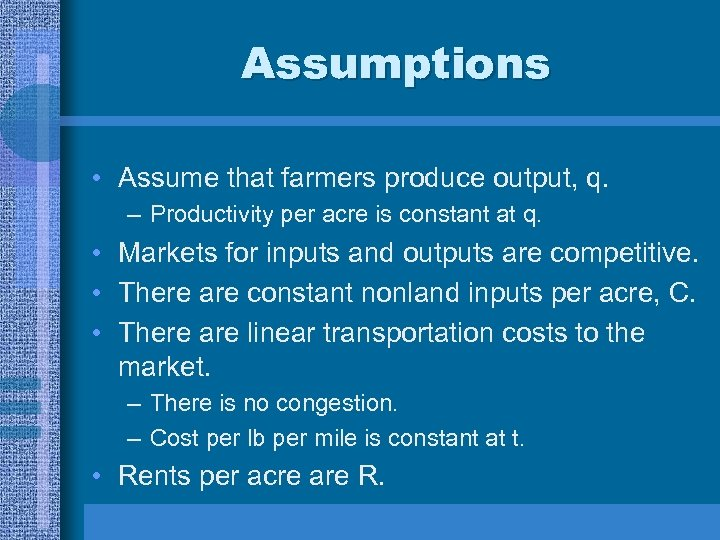Assumptions • Assume that farmers produce output, q. – Productivity per acre is constant at q. • Markets for inputs and outputs are competitive. • There are constant nonland inputs per acre, C. • There are linear transportation costs to the market. – There is no congestion. – Cost per lb per mile is constant at t. • Rents per acre are R.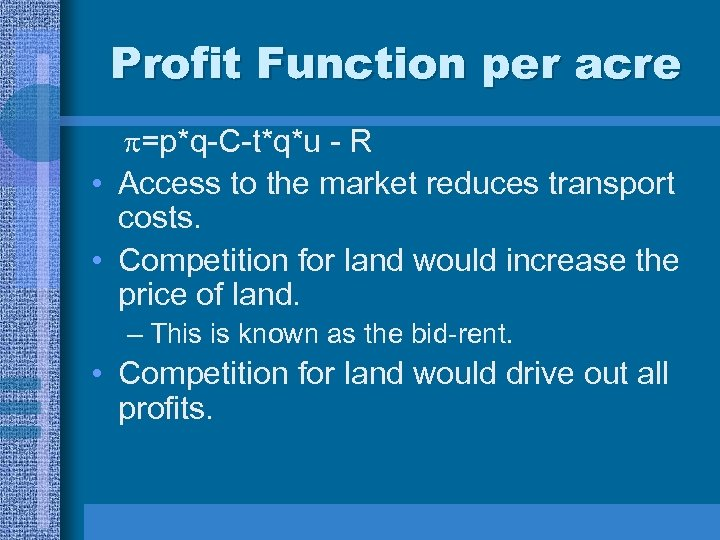Profit Function per acre =p*q-C-t*q*u - R • Access to the market reduces transport costs. • Competition for land would increase the price of land. – This is known as the bid-rent. • Competition for land would drive out all profits.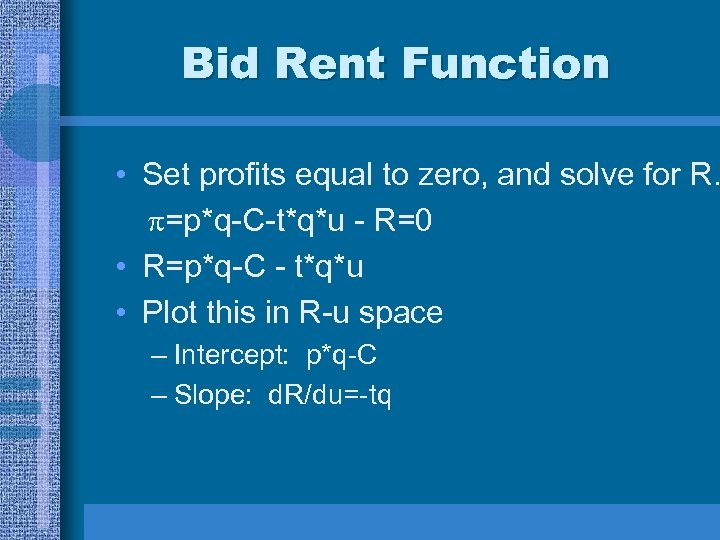Bid Rent Function • Set profits equal to zero, and solve for R. =p*q-C-t*q*u - R=0 • R=p*q-C - t*q*u • Plot this in R-u space – Intercept: p*q-C – Slope: d. R/du=-tq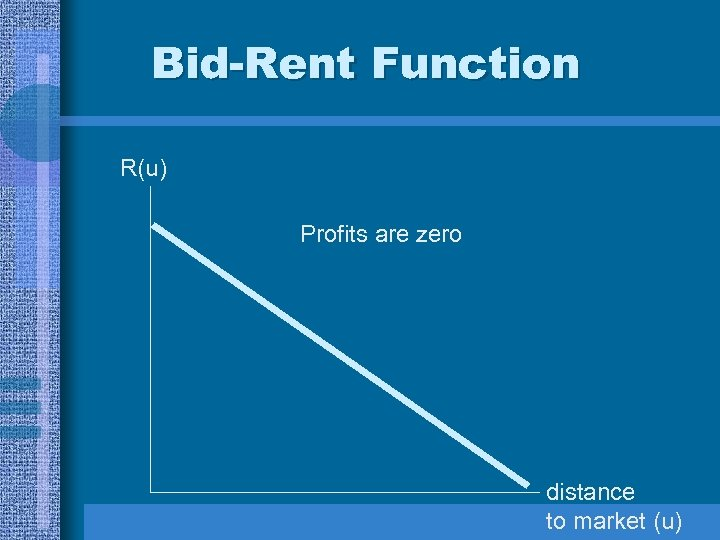Bid-Rent Function R(u) Profits are zero distance to market (u)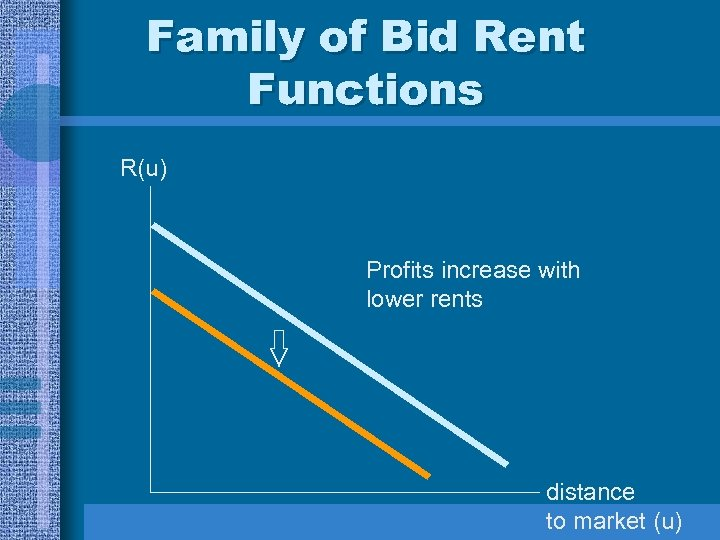Family of Bid Rent Functions R(u) Profits increase with lower rents distance to market (u)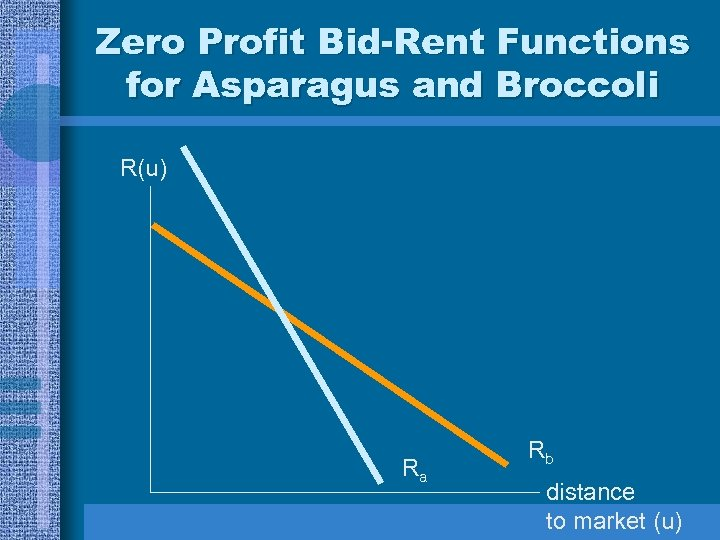Zero Profit Bid-Rent Functions for Asparagus and Broccoli R(u) Ra Rb distance to market (u)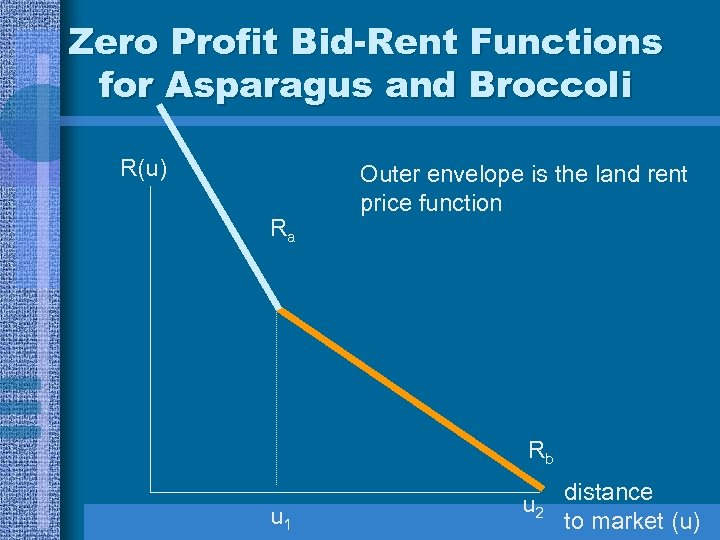Zero Profit Bid-Rent Functions for Asparagus and Broccoli R(u) Ra Outer envelope is the land rent price function Rb u 1 u 2 distance to market (u)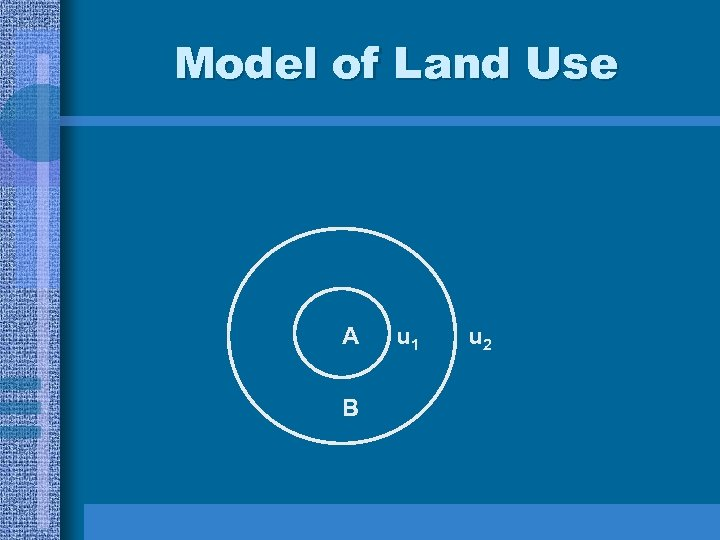Model of Land Use A B u 1 u 2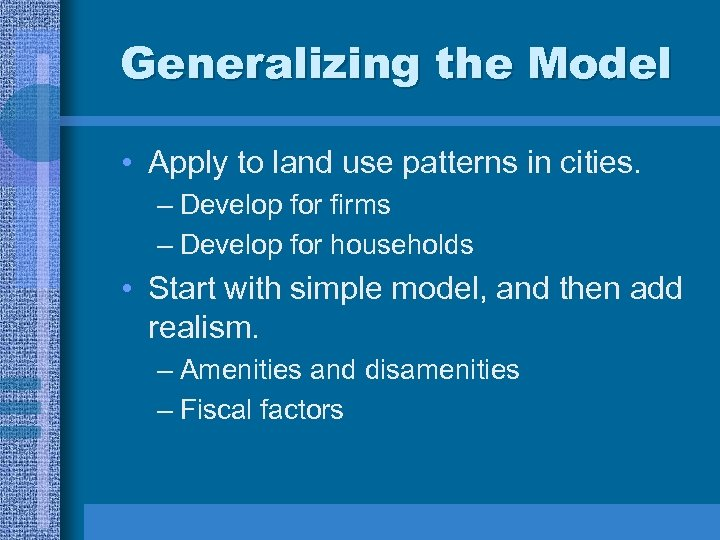Generalizing the Model • Apply to land use patterns in cities. – Develop for firms – Develop for households • Start with simple model, and then add realism. – Amenities and disamenities – Fiscal factors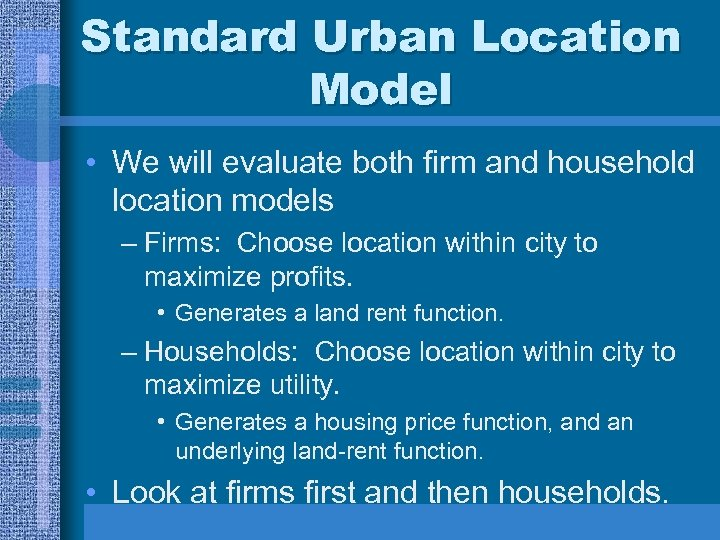Standard Urban Location Model • We will evaluate both firm and household location models – Firms: Choose location within city to maximize profits. • Generates a land rent function. – Households: Choose location within city to maximize utility. • Generates a housing price function, and an underlying land-rent function. • Look at firms first and then households.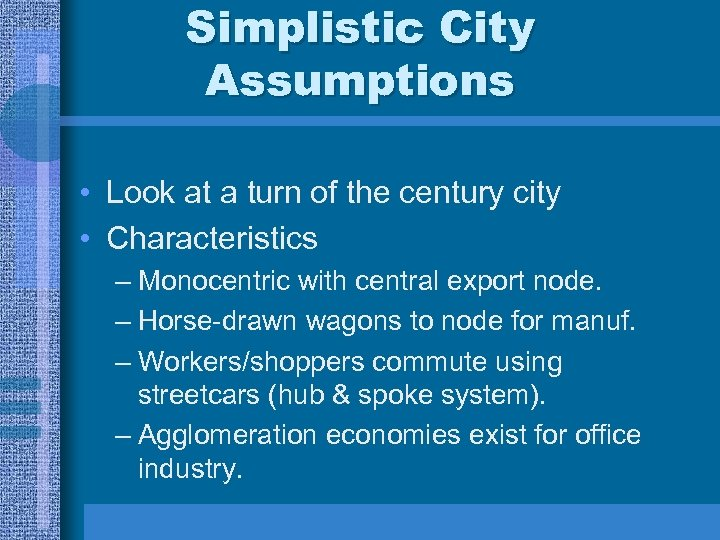Simplistic City Assumptions • Look at a turn of the century city • Characteristics – Monocentric with central export node. – Horse-drawn wagons to node for manuf. – Workers/shoppers commute using streetcars (hub & spoke system). – Agglomeration economies exist for office industry.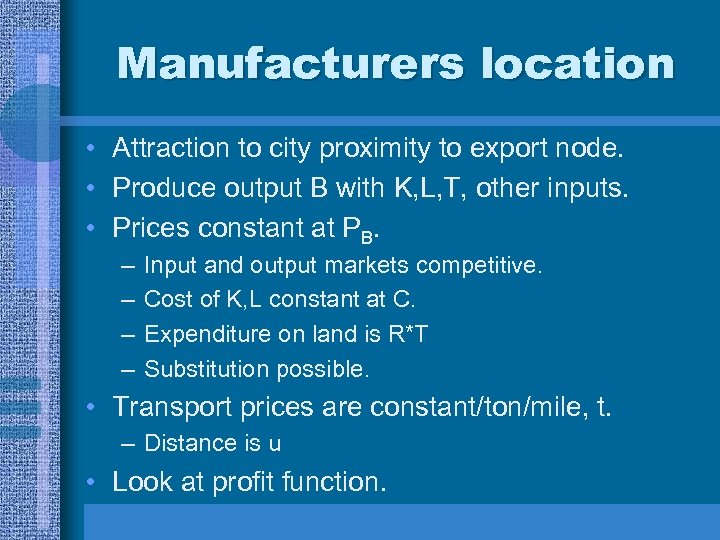Manufacturers location • Attraction to city proximity to export node. • Produce output B with K, L, T, other inputs. • Prices constant at PB. – – Input and output markets competitive. Cost of K, L constant at C. Expenditure on land is R*T Substitution possible. • Transport prices are constant/ton/mile, t. – Distance is u • Look at profit function.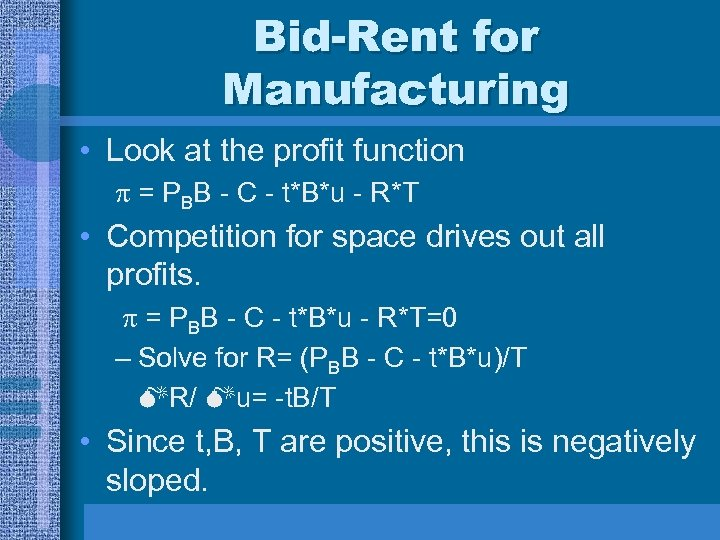Bid-Rent for Manufacturing • Look at the profit function = PBB - C - t*B*u - R*T • Competition for space drives out all profits. = PBB - C - t*B*u - R*T=0 – Solve for R= (PBB - C - t*B*u)/T R/ u= -t. B/T • Since t, B, T are positive, this is negatively sloped.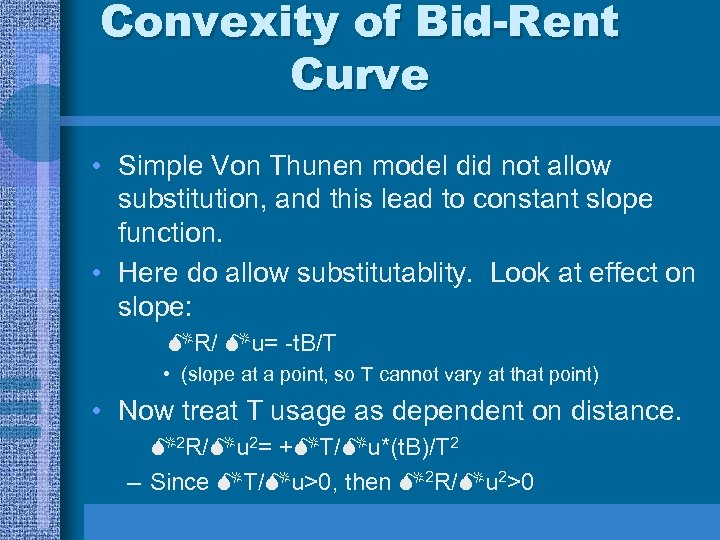Convexity of Bid-Rent Curve • Simple Von Thunen model did not allow substitution, and this lead to constant slope function. • Here do allow substitutablity. Look at effect on slope: R/ u= -t. B/T • (slope at a point, so T cannot vary at that point) • Now treat T usage as dependent on distance. 2 R/ u 2= + T/ u*(t. B)/T 2 – Since T/ u>0, then 2 R/ u 2>0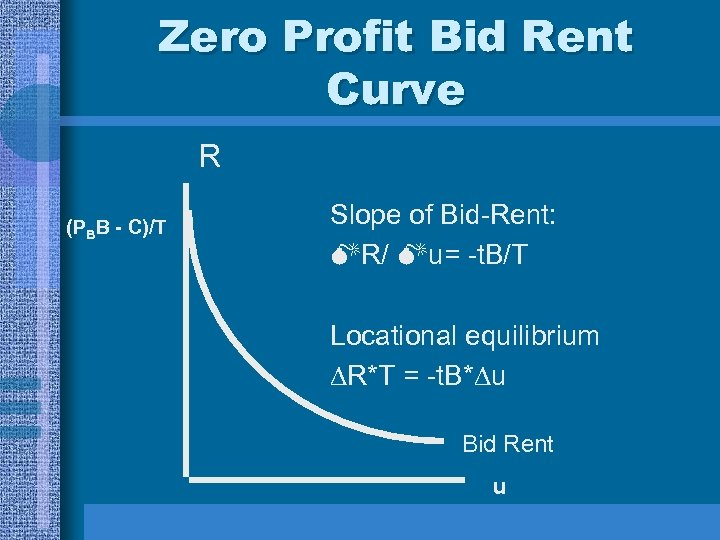Zero Profit Bid Rent Curve R (PBB - C)/T Slope of Bid-Rent: R/ u= -t. B/T Locational equilibrium R*T = -t. B* u Bid Rent u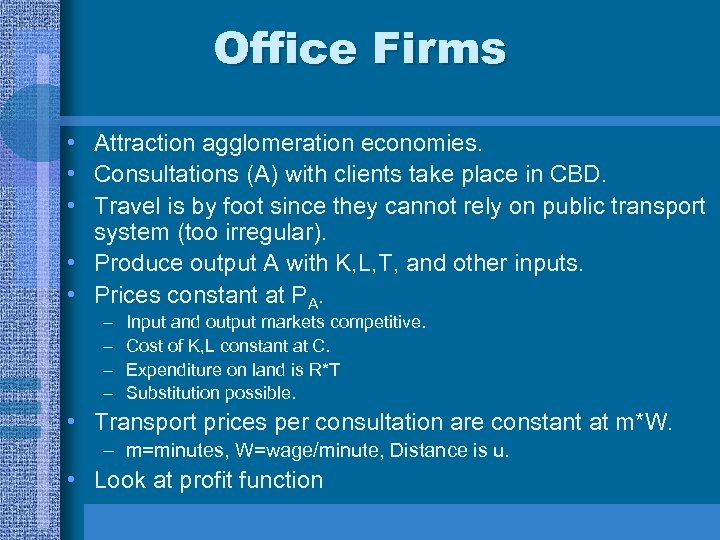Office Firms • Attraction agglomeration economies. • Consultations (A) with clients take place in CBD. • Travel is by foot since they cannot rely on public transport system (too irregular). • Produce output A with K, L, T, and other inputs. • Prices constant at PA. – – Input and output markets competitive. Cost of K, L constant at C. Expenditure on land is R*T Substitution possible. • Transport prices per consultation are constant at m*W. – m=minutes, W=wage/minute, Distance is u. • Look at profit function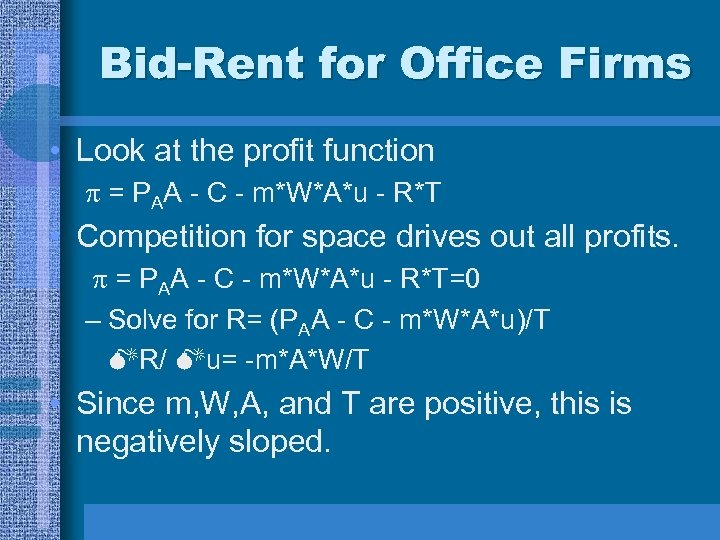Bid-Rent for Office Firms • Look at the profit function = PAA - C - m*W*A*u - R*T • Competition for space drives out all profits. = PAA - C - m*W*A*u - R*T=0 – Solve for R= (PAA - C - m*W*A*u)/T R/ u= -m*A*W/T • Since m, W, A, and T are positive, this is negatively sloped.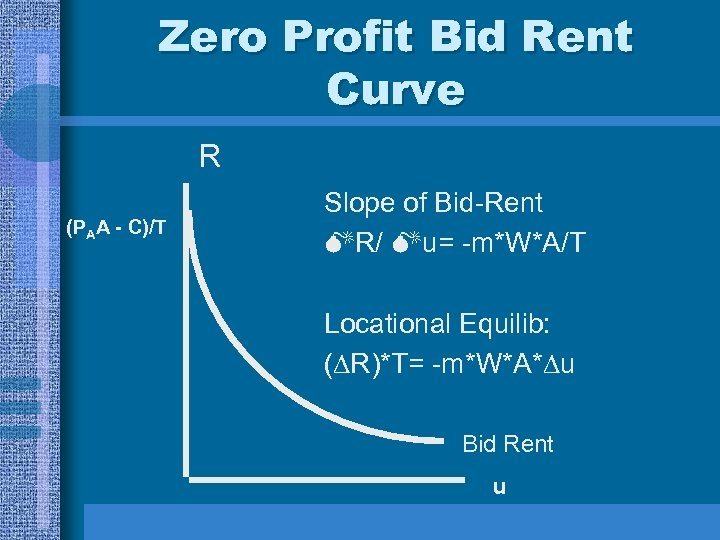Zero Profit Bid Rent Curve R (PAA - C)/T Slope of Bid-Rent R/ u= -m*W*A/T Locational Equilib: ( R)*T= -m*W*A* u Bid Rent u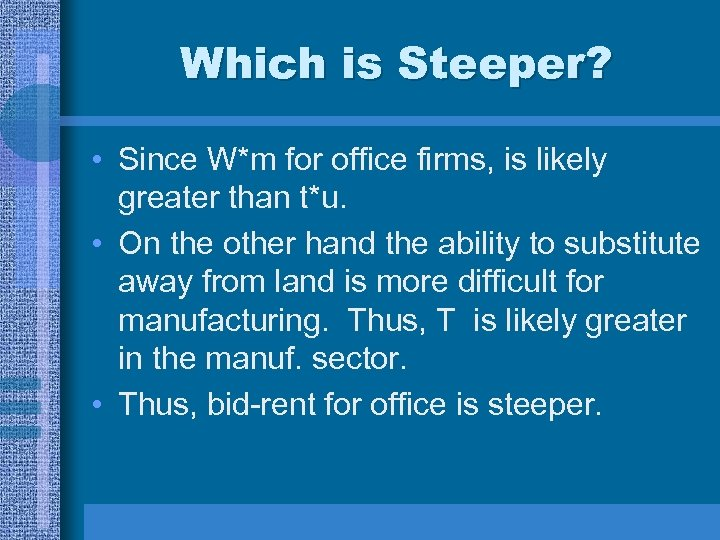Which is Steeper? • Since W*m for office firms, is likely greater than t*u. • On the other hand the ability to substitute away from land is more difficult for manufacturing. Thus, T is likely greater in the manuf. sector. • Thus, bid-rent for office is steeper.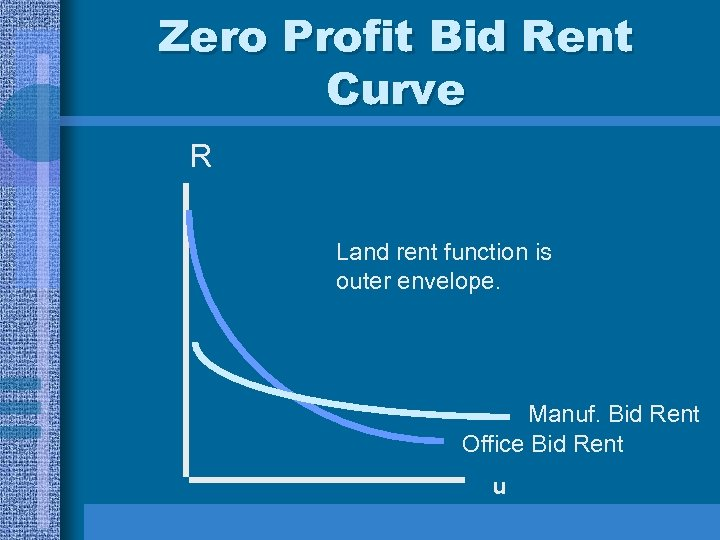Zero Profit Bid Rent Curve R Land rent function is outer envelope. Manuf. Bid Rent Office Bid Rent u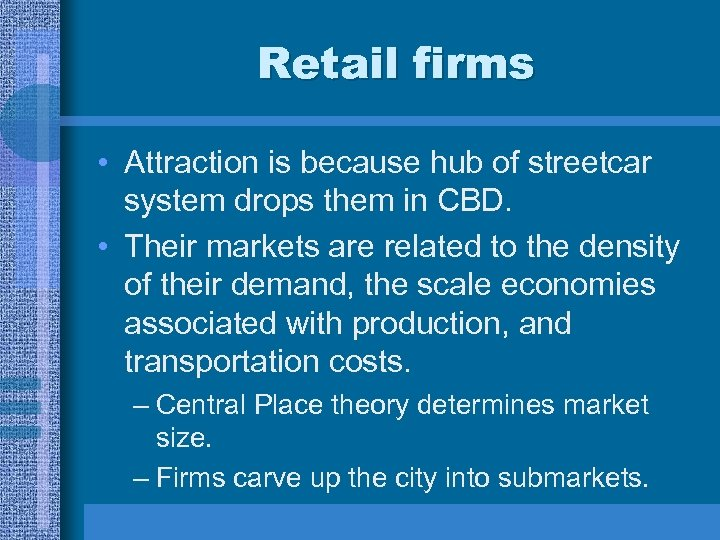Retail firms • Attraction is because hub of streetcar system drops them in CBD. • Their markets are related to the density of their demand, the scale economies associated with production, and transportation costs. – Central Place theory determines market size. – Firms carve up the city into submarkets.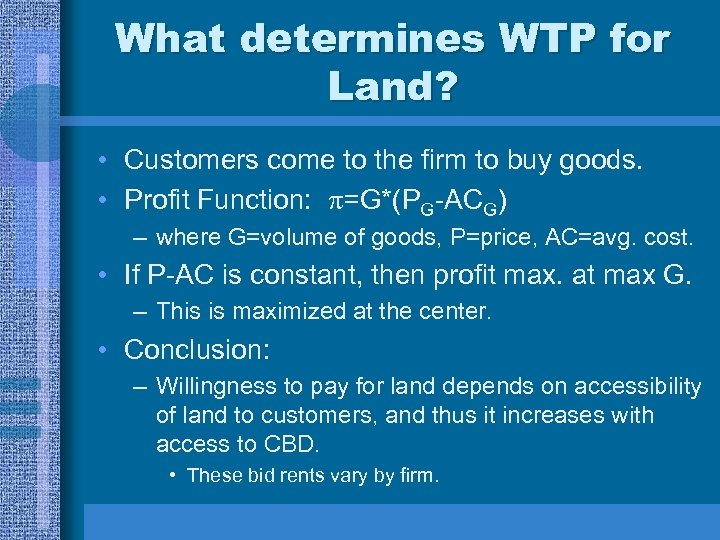What determines WTP for Land? • Customers come to the firm to buy goods. • Profit Function: =G*(PG-ACG) – where G=volume of goods, P=price, AC=avg. cost. • If P-AC is constant, then profit max. at max G. – This is maximized at the center. • Conclusion: – Willingness to pay for land depends on accessibility of land to customers, and thus it increases with access to CBD. • These bid rents vary by firm.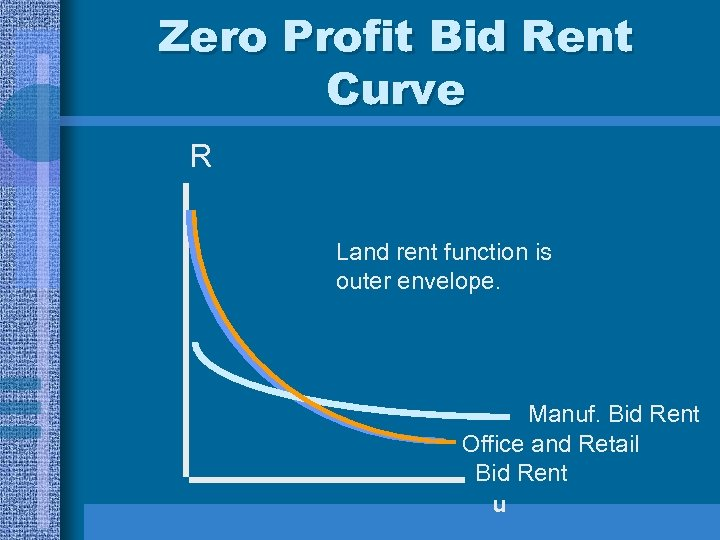Zero Profit Bid Rent Curve R Land rent function is outer envelope. Manuf. Bid Rent Office and Retail Bid Rent u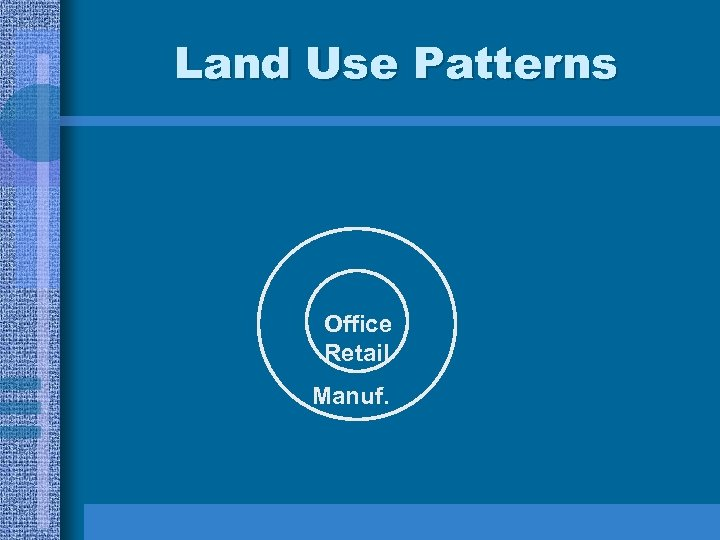Land Use Patterns Office O Retail Manuf.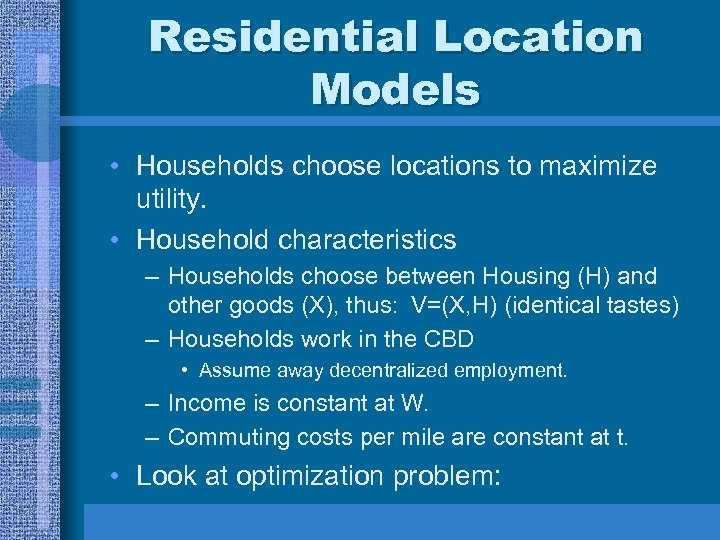Residential Location Models • Households choose locations to maximize utility. • Household characteristics – Households choose between Housing (H) and other goods (X), thus: V=(X, H) (identical tastes) – Households work in the CBD • Assume away decentralized employment. – Income is constant at W. – Commuting costs per mile are constant at t. • Look at optimization problem: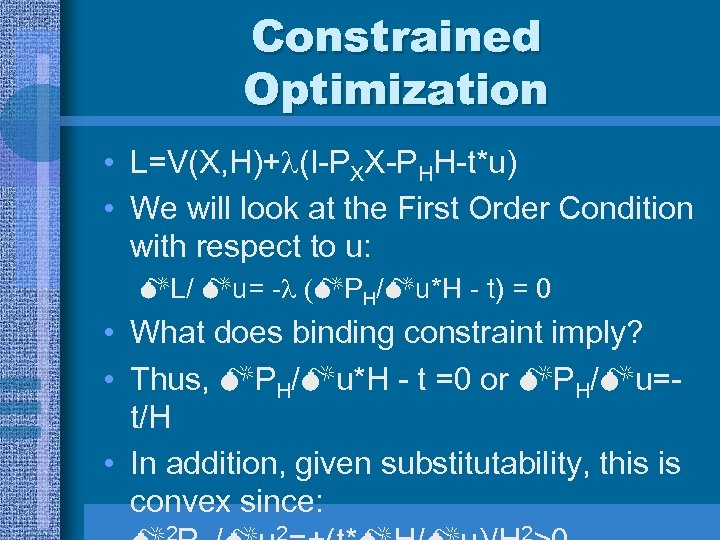Constrained Optimization • L=V(X, H)+ (I-PXX-PHH-t*u) • We will look at the First Order Condition with respect to u: L/ u= - PH/ u*H - t) = 0 • What does binding constraint imply? • Thus, PH/ u*H - t =0 or PH/ u=t/H • In addition, given substitutability, this is convex since: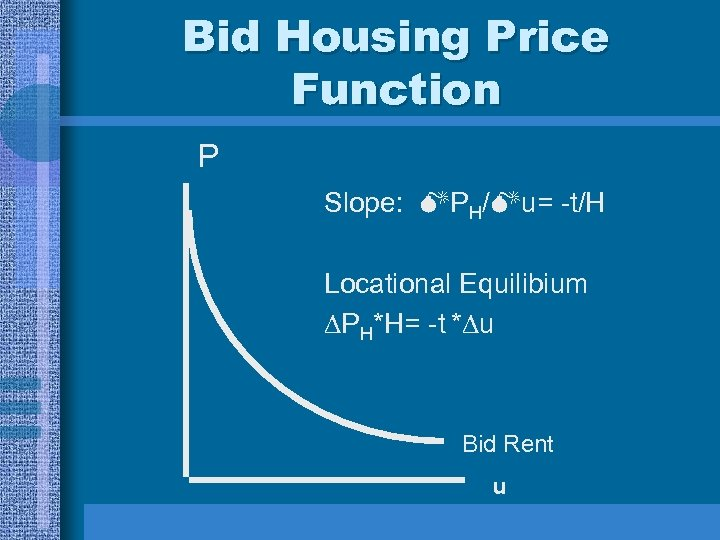Bid Housing Price Function P Slope: PH/ u= -t/H Locational Equilibium PH*H= -t * u Bid Rent u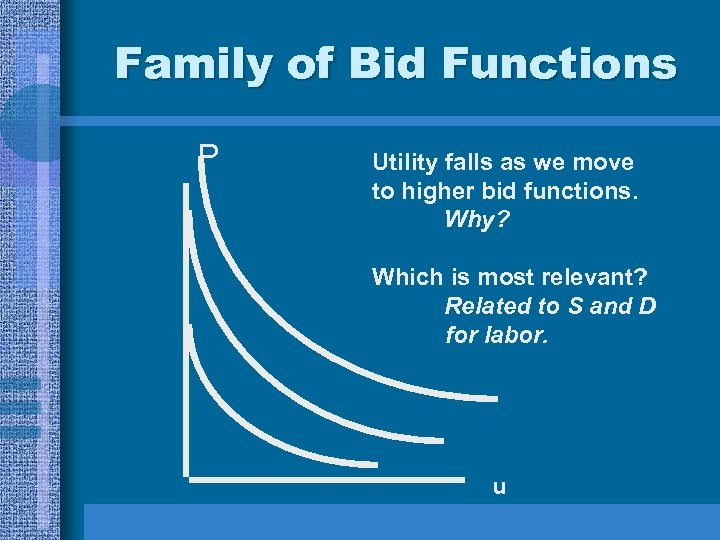Family of Bid Functions P Utility falls as we move to higher bid functions. Why? Which is most relevant? Related to S and D for labor. u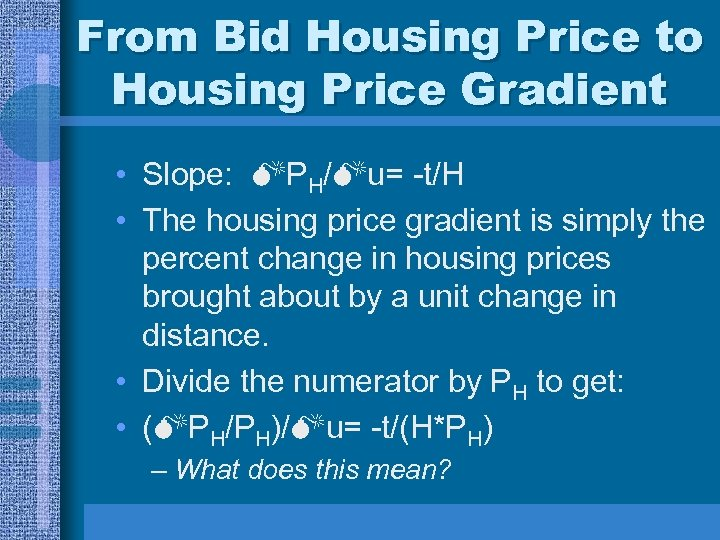From Bid Housing Price to Housing Price Gradient • Slope: PH/ u= -t/H • The housing price gradient is simply the percent change in housing prices brought about by a unit change in distance. • Divide the numerator by PH to get: • ( PH/PH)/ u= -t/(H*PH) – What does this mean?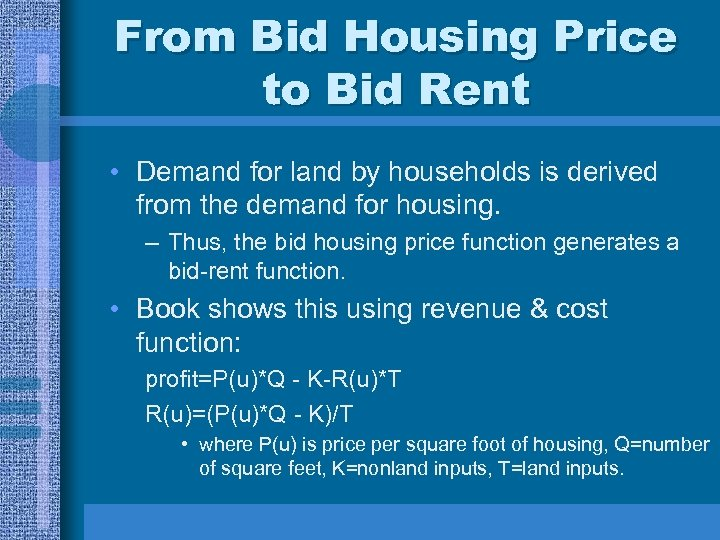From Bid Housing Price to Bid Rent • Demand for land by households is derived from the demand for housing. – Thus, the bid housing price function generates a bid-rent function. • Book shows this using revenue & cost function: profit=P(u)*Q - K-R(u)*T R(u)=(P(u)*Q - K)/T • where P(u) is price per square foot of housing, Q=number of square feet, K=nonland inputs, T=land inputs.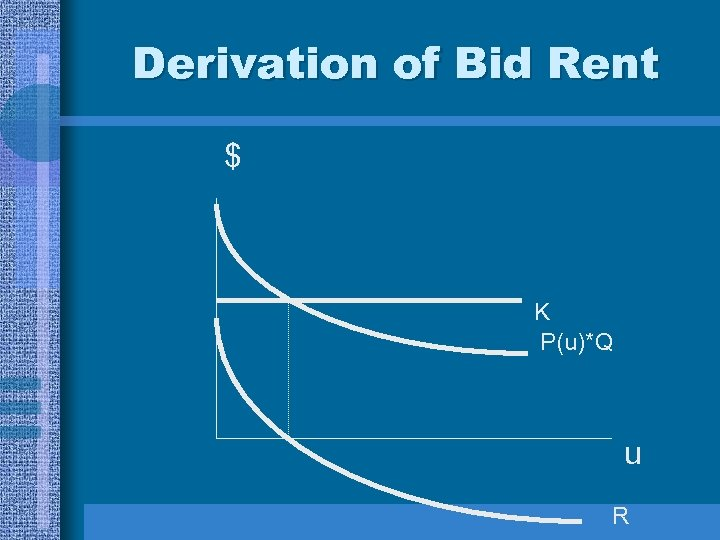Derivation of Bid Rent \$ K P(u)*Q u R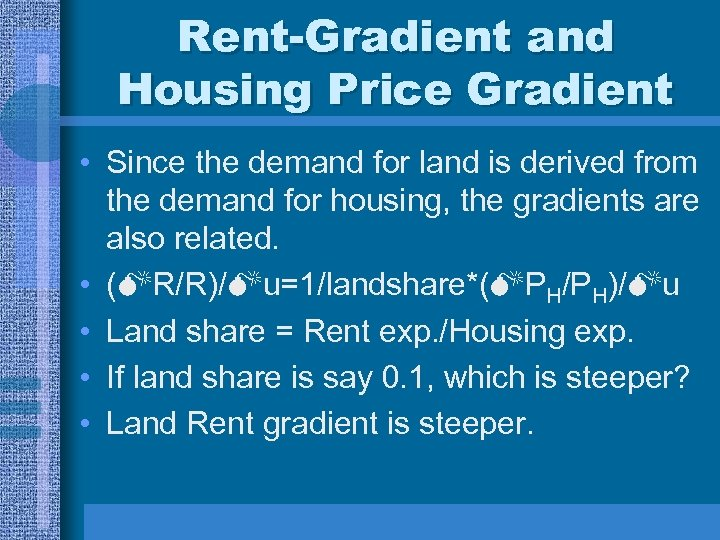Rent-Gradient and Housing Price Gradient • Since the demand for land is derived from the demand for housing, the gradients are also related. • ( R/R)/ u=1/landshare*( PH/PH)/ u • Land share = Rent exp. /Housing exp. • If land share is say 0. 1, which is steeper? • Land Rent gradient is steeper.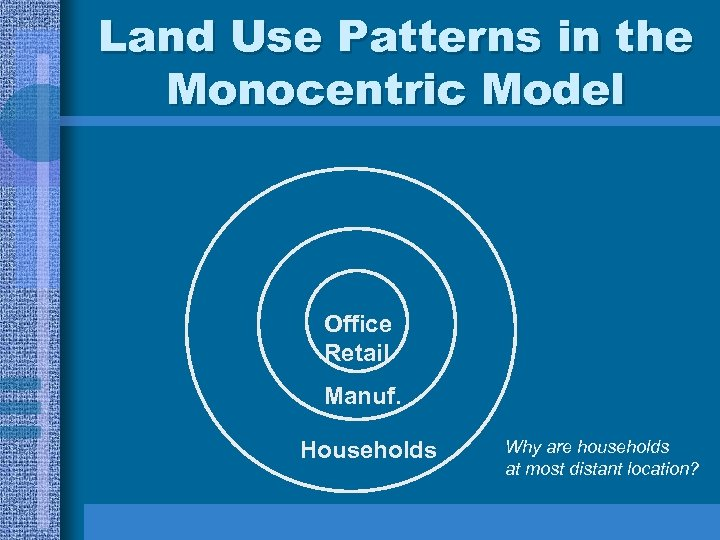Land Use Patterns in the Monocentric Model Office O Retail Manuf. Households Why are households at most distant location?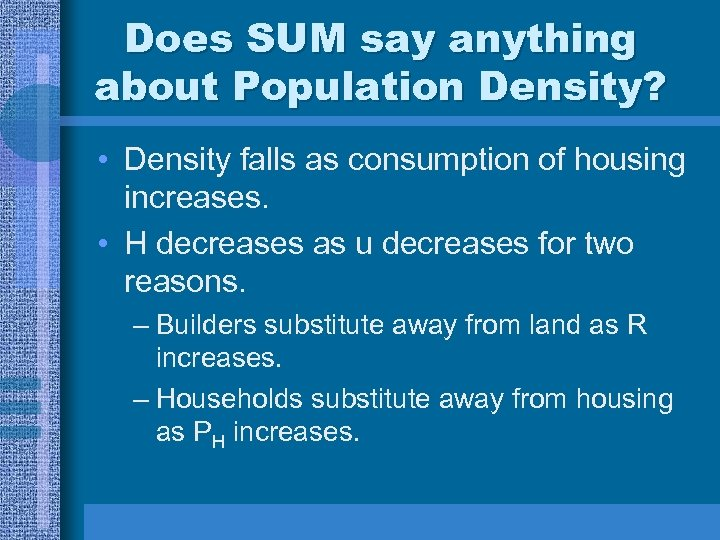Does SUM say anything about Population Density? • Density falls as consumption of housing increases. • H decreases as u decreases for two reasons. – Builders substitute away from land as R increases. – Households substitute away from housing as PH increases.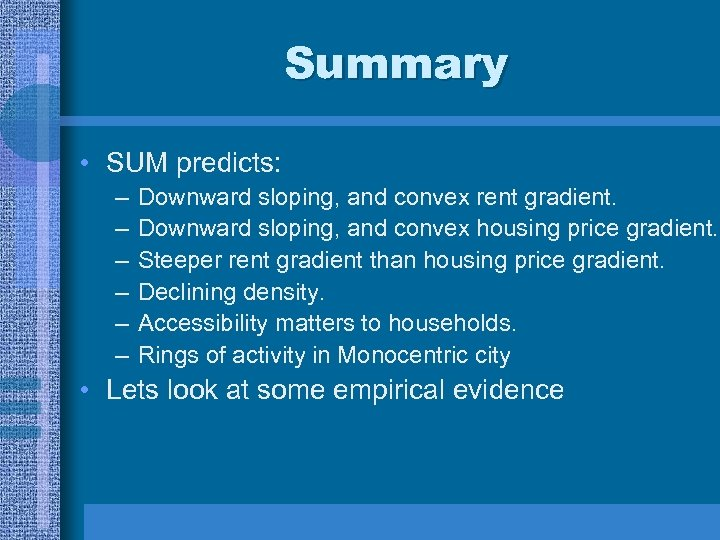Summary • SUM predicts: – – – Downward sloping, and convex rent gradient. Downward sloping, and convex housing price gradient. Steeper rent gradient than housing price gradient. Declining density. Accessibility matters to households. Rings of activity in Monocentric city • Lets look at some empirical evidence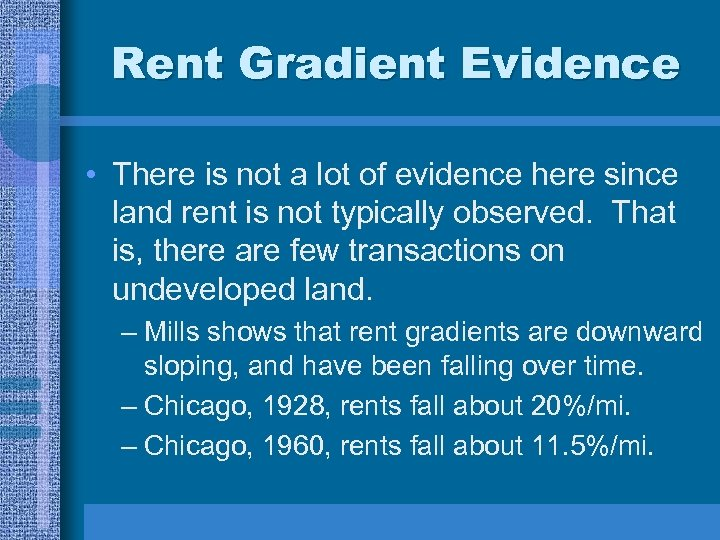Rent Gradient Evidence • There is not a lot of evidence here since land rent is not typically observed. That is, there are few transactions on undeveloped land. – Mills shows that rent gradients are downward sloping, and have been falling over time. – Chicago, 1928, rents fall about 20%/mi. – Chicago, 1960, rents fall about 11. 5%/mi.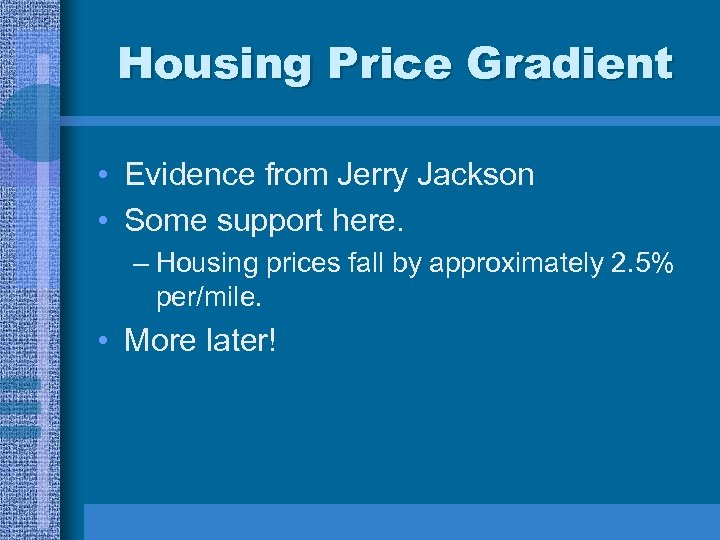Housing Price Gradient • Evidence from Jerry Jackson • Some support here. – Housing prices fall by approximately 2. 5% per/mile. • More later!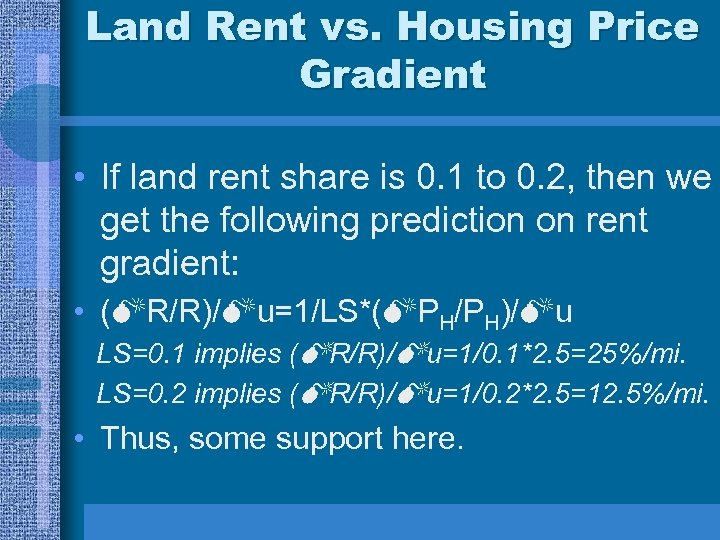Land Rent vs. Housing Price Gradient • If land rent share is 0. 1 to 0. 2, then we get the following prediction on rent gradient: • ( R/R)/ u=1/LS*( PH/PH)/ u LS=0. 1 implies ( R/R)/ u=1/0. 1*2. 5=25%/mi. LS=0. 2 implies ( R/R)/ u=1/0. 2*2. 5=12. 5%/mi. • Thus, some support here.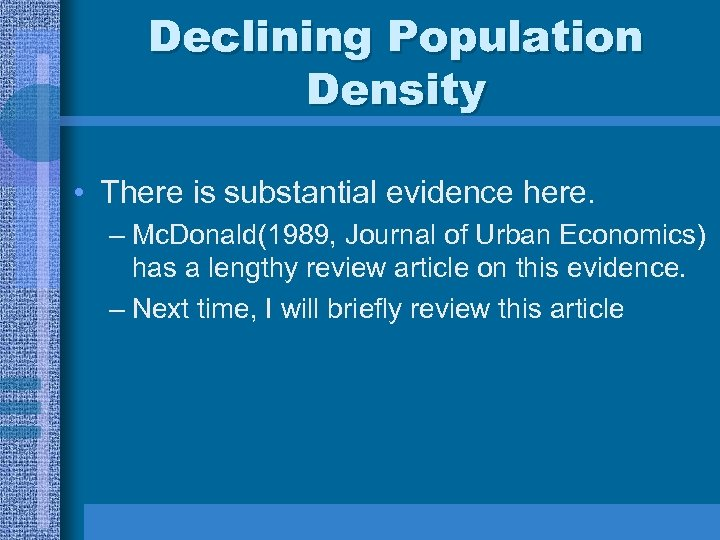Declining Population Density • There is substantial evidence here. – Mc. Donald(1989, Journal of Urban Economics) has a lengthy review article on this evidence. – Next time, I will briefly review this article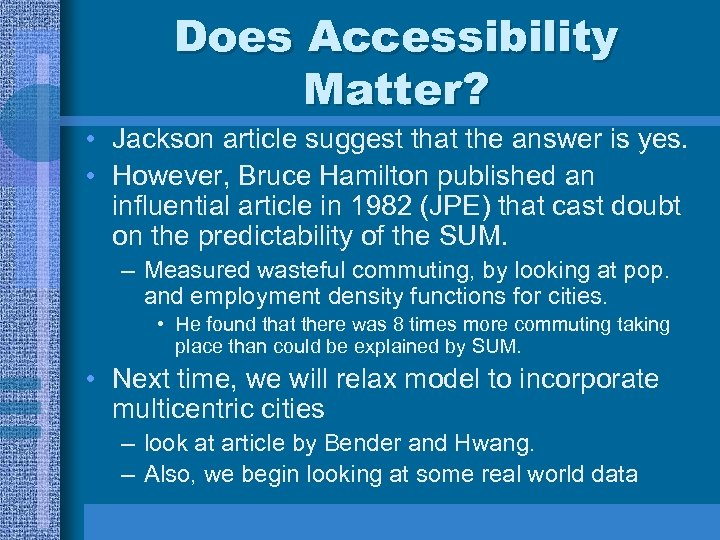Does Accessibility Matter? • Jackson article suggest that the answer is yes. • However, Bruce Hamilton published an influential article in 1982 (JPE) that cast doubt on the predictability of the SUM. – Measured wasteful commuting, by looking at pop. and employment density functions for cities. • He found that there was 8 times more commuting taking place than could be explained by SUM. • Next time, we will relax model to incorporate multicentric cities – look at article by Bender and Hwang. – Also, we begin looking at some real world data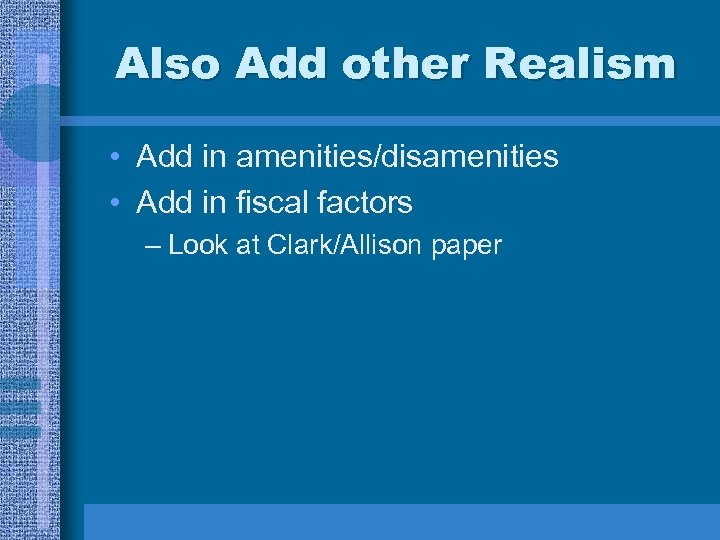Also Add other Realism • Add in amenities/disamenities • Add in fiscal factors – Look at Clark/Allison paper